# 28 Results

View
Selected filters:
• Tmm0102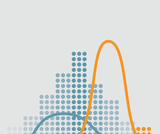Rating

Introductory statistics course developed through the Ohio Department of Higher Education OER Innovation Grant. The course is part of the Ohio Transfer Module and is also named TMM010. For more information about credit transfer between Ohio colleges and universities please visit: www.ohiohighered.org/transfer.Team LeadKameswarrao Casukhela                     Ohio State University – LimaContent ContributorsEmily Dennett                                       Central Ohio Technical CollegeSara Rollo                                            North Central State CollegeNicholas Shay                                      Central Ohio Technical CollegeChan Siriphokha                                   Clark State Community CollegeLibrarianJoy Gao                                                Ohio Wesleyan UniversityReview TeamAlice Taylor                                           University of Rio GrandeJim Cottrill                                             Ohio Dominican University

Subject:
Mathematics
Statistics and Probability
Material Type:
Full Course
Provider:
Ohio Open Ed Collaborative
04/17/2018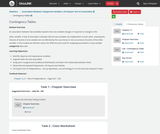Conditional Remix & Share Permitted
CC BY-NC
Rating

An association between two variables explains how one variable changes in response to changes in the other variable. A lack of association indicates that the two variables are independent of each other, meaning the chances of events of one variable are not affected by the occurrence or non-occurrence of events of the other variable. In this module we will learn about the different tools used for analyzing associations in two-variable categorical data sets.Learning Objectives:Identify response and explanatory variablesOrganize data into two-way tablesStudy joint, marginal and conditional distributions and learn the relationship between themObserved and expected frequencies, Chi-Square test statisticChi-Square test of independence – set up hypothesis, use technology to run the test and interpret P-valueTextbook Material -  ·         Chapter 11.3 – Test of Independence – Pages 627 - 632

Subject:
Statistics and Probability
Material Type:
Module
Provider:
Ohio Open Ed Collaborative
07/03/2018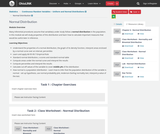Conditional Remix & Share Permitted
CC BY-NC
Rating

Many inferential procedures assume that variable(s) under study follow a normal distribution in the population. In this module we will study properties of this distribution and learn how to calculate important measures that would be useful later in inference.Learning Objectives:Understand the properties of a normal distribution, the graph of its density function, interpret areas enclosed by a normal curve over an interval, percentilesLearn and apply 68-95-99.7 Empirical RuleStandard normal distribution, z-scores and standard normal tableCompute areas under the normal curve and interpret the resultsCompute percentiles and interpret the resultsCalculate cut-off values of the variable to cover middle p% of the distributionHow normal is a population distribution - Learn how to infer that the population distribution of the variable is normal – set up hypothesis, use normal probability plot, Anderson-Darling normality test, interpret p-value of the testChapter 6 – Normal Distribution – Pages 361 - 375Suggested Exercises – Chapter 6 – Odds 60 through 80

Subject:
Statistics and Probability
Material Type:
Module
Provider:
Ohio Open Ed Collaborative
07/03/2018Remix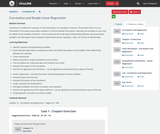Conditional Remix & Share Permitted
CC BY-NC
Rating

Sometimes it is difficult to measure or find information on a variable of interest. The problem then is to use information from easily measurable variables to find the needed information. Naturally, the variables to use must be related to the variable of interest. In this module we will study about relationships between two quantitative variables. We will explore some standard mathematical (linear, quadratic, cubic, etc.) forms of relationships.Learning Objectives:Identify response and explanatory variablesGiven bivariate data make a scatterplot of data and predict the pattern and strength of the relationship between the variablesLinear relationshipDefine correlation, study its properties and use themFind correlation for a bivariate data and interpret the resultsInterpret the square of the correlationTest for the significance of correlation – set up hypothesis and interpret the p-value of the testLinear relationship – Estimate the linear relationship between the two variables.Interpret slope and intercept.Interpret the square of the correlationStudy residuals and residual plots,Distinguish between the terms correlation and causationTest for the significance of the slope coefficient – set up hypothesis and interpret the p-value of the test.Study quadratic and other non-linear models.Textbook Material -  Chapter 12 – Correlation and Regression – Pages 673 - 699

Subject:
Statistics and Probability
Material Type:
Module
Provider:
Ohio Open Ed Collaborative
Author:
Kameswarrao Casukhela
09/06/2018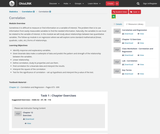Conditional Remix & Share Permitted
CC BY-NC
Rating

Sometimes it is difficult to measure or find information on a variable of interest. The problem then is to use information from easily measurable variables to find the needed information. Naturally, the variables to use must be related to the variable of interest. In this module we will study about relationships between two quantitative variables. We will explore some standard mathematical (linear, quadratic, cubic, etc.) forms of relationships.Learning Objectives:Identify response and explanatory variablesGiven bivariate data make a scatterplot of data and predict the pattern and strength of the relationship between the variablesLinear relationshipDefine correlation, study its properties and use themFind correlation for a bivariate data and interpret the resultsInterpret the square of the correlationTest for the significance of correlation – set up hypothesis and interpret the p-value of the testLinear relationship – Estimate the linear relationship between the two variables.Interpret slope and intercept.Interpret the square of the correlationStudy residuals and residual plots,Distinguish between the terms correlation and causationTest for the significance of the slope coefficient – set up hypothesis and interpret the p-value of the test.Study quadratic and other non-linear models.Textbook Material -  Chapter 12 – Correlation and Regression – Pages 673 - 699

Subject:
Statistics and Probability
Material Type:
Module
Provider:
Ohio Open Ed Collaborative
07/03/2018Conditional Remix & Share Permitted
CC BY-NC
Rating

This module contains a template for course final project which ties up different concepts taught in an intro stats course. Student are encouraged to seek problems that could be answered using relationships between variables. Students need to collect, summarize, visually represent and explore relationships using data.

Subject:
Statistics and Probability
Material Type:
Module
Provider:
Ohio Open Ed Collaborative
Author:
Kameswarrao Casukhela
Sara Rollo
08/29/2018Rating

The study of a population, a process or a relationship of interest begins with collecting relevant data from representative samples. A good understanding of the key terms will help us design appropriate statistical analysis of the collected data.Learning Objectives:Distinguish the terms – population, parameter, sample, statistics, and other key terms.Distinguish between different types of statistical studies – observational, experimental, retrospective or prospectiveLearn about different levels of measurement, data types, and variablesLearn about different ways of collecting data – Sampling Methods and ExperimentsIdentify sources of measurement bias and variability

Subject:
Mathematics
Statistics and Probability
Material Type:
Unit of Study
Provider:
Ohio Open Ed Collaborative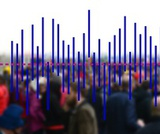Conditional Remix & Share Permitted
CC BY-NC
Rating

The study of a population, a process or a relationship of interest begins with collecting relevant data from representative samples. A good understanding of the key terms will help us design appropriate statistical analysis of the collected data.Learning Objectives:Distinguish between the terms – population, parameter, sample, statistics, and other key terms.Distinguish between different types of statistical studies – observational, experimental, retrospective or prospectiveLearn about different levels of measurement, data types, and variablesLearn about different ways of collecting data – Sampling Methods and ExperimentsIdentify sources of measurement bias and variabilityTextbook Material: Chapter 1, Sampling & DataSuggested Homework AssignmentChapter 1 – Pages 47 – 60 - Problems 42 to 62, 65, 67, 73, 74, 75, 78, 87, 91

Subject:
Statistics and Probability
Material Type:
Module
Provider:
Ohio Open Ed Collaborative
06/07/2018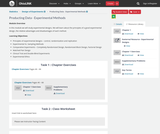Conditional Remix & Share Permitted
CC BY-NC
Rating

In this module we will study experimental designs. We will learn about the principles of a good experimental design, the relative advantages and disadvantages of each method.Learning Objectives:Principles of experimental designs – control, randomization and replicationExperimental Vs. Sampling MethodsComparative Experiments – Completely Randomized Design, Randomized Block Design, Factorial DesignMatched Pair DesignClinical Trial and Double-Blind ExperimentsExperimental Ethics

Subject:
Statistics and Probability
Material Type:
Module
Provider:
Ohio Open Ed Collaborative
07/02/2018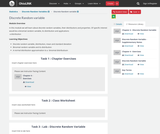Conditional Remix & Share Permitted
CC BY-NC
Rating

In this module we will learn about discrete random variables, their distributions and properties. Of specific interest would be a binomial random variable, its distribution and applications.Learning Objectives:Discrete random variable, distribution, mean and standard deviationBinomial random variable and its distributionTextbook Material -  Chapter 4 – Probability Topics – Pages 239 - 257Suggested Homework:Discrete Random Variable - 69, 70, 74, 75, 76, 78, 96, 102Binomial Distribution – Odds 88 - 111

Subject:
Statistics and Probability
Material Type:
Module
Provider:
Ohio Open Ed Collaborative
07/03/2018Conditional Remix & Share Permitted
CC BY-NC
Rating

In this module we will explore the concept of a confidence interval to estimate an unknown population parameter. Specifically, we will obtain confidence interval estimates for unknown population mean and for unknown population proportion. We will also find how large a sample we would require to estimate these parameters for any given amount of accuracy and confidence level.Learning Objectives:Construct and interpret a confidence intervalStudent’s t-distributionStandard error and margin of errorSample size estimationTextbook Material -  Chapter 8 – Confidence Intervals – pages 439 - 463Suggested Exercises – Odds 97 – 134

Subject:
Statistics and Probability
Material Type:
Module
Provider:
Ohio Open Ed Collaborative